Question

# Suppose Tori has an unfair coin which lands on Tails with probability 0.28 when flipped. If...

Suppose Tori has an unfair coin which lands on Tails with probability 0.28 when flipped. If she flips the coin 10 times, find each of the following:

Question 2 options:

 The standard deviation of the number of Tails P(Exactly 1 Tail) P(More than 3 Tails) The mean number of Tails P(At least 5 Tails) P(No more than 3 Tails) P(Less than or equal to 2 Tails) P(Exactly 4 Tails) P(At least 1 Tail) P(No Tails)
 1 0.1798 2 0.7021 3 2.8 4 0.1181 5 0.1456 6 0.0374 7 0.9626 8 0.2979 9 0.4378 10 1.42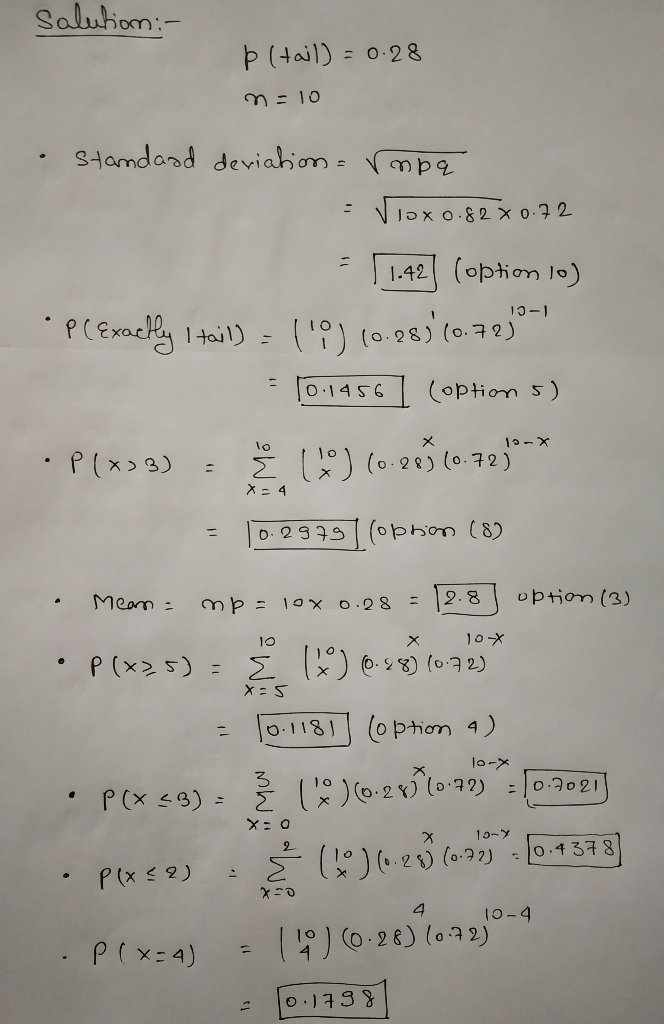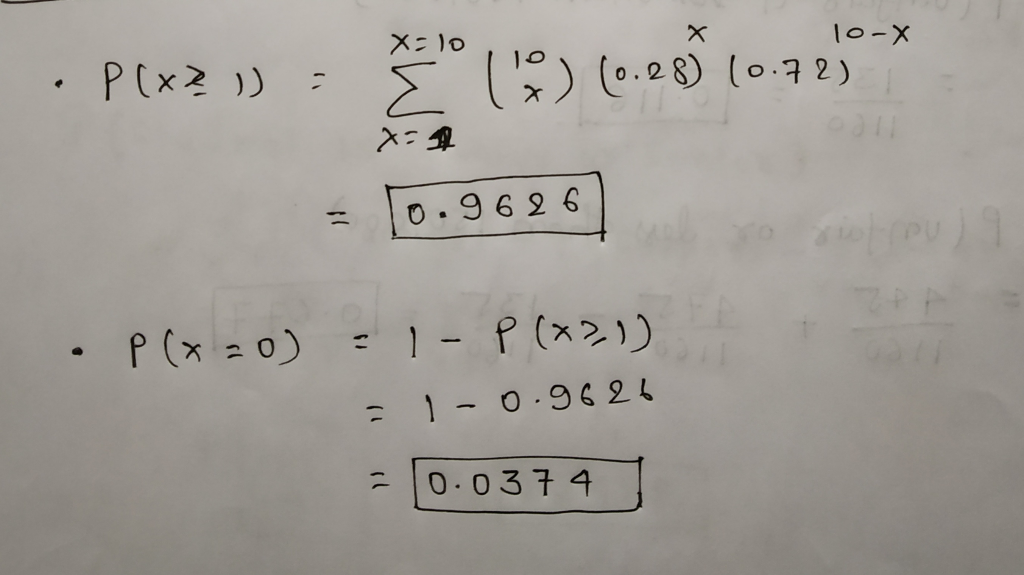#### Earn Coins

Coins can be redeemed for fabulous gifts.

Similar Homework Help Questions
• ### Question 2 (2 points) Suppose Tori has an unfair coin which lands on Tails with probability...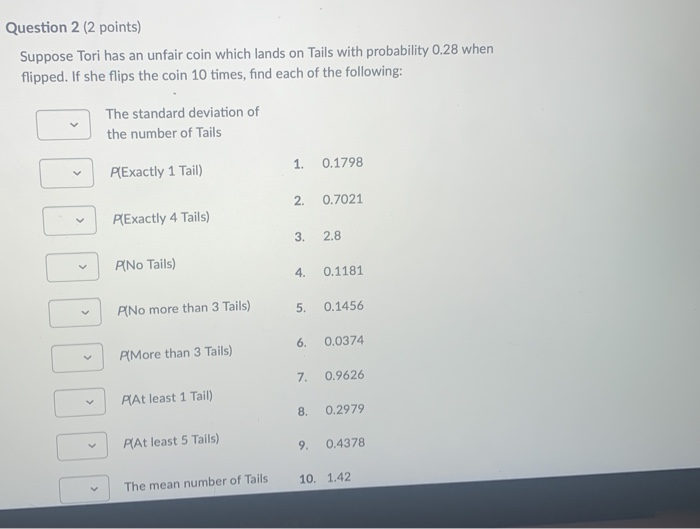Question 2 (2 points) Suppose Tori has an unfair coin which lands on Tails with probability 0.28 when flipped. If she flips the coin 10 times, find each of the following: The standard deviation of the number of Tails PExactly 1 Tail) 1. 0.1798 2. 0.7021 PExactly 4 Tails) 3. 2.8 PiNo Tails) 4 0.1181 P[No more than 3 Tails) 5. 0.1456 PlMore than 3 Tails) 6. 0.0374 7. 0.9626 PlAt least 1 Tail) 8. 0.2979 PAt least 5 Tails)...

• ### when coin 2 is flipped it lands on heads with When coin 1 is flipped, it...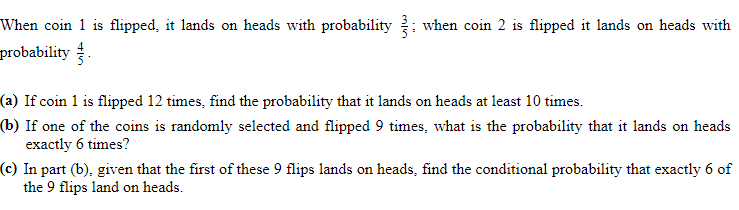when coin 2 is flipped it lands on heads with When coin 1 is flipped, it lands on heads with probability probability (a) If coin 1 is flipped 12 times, find the probability that it lands on heads at least 10 times. (b) If one of the coins is randomly selected and flipped 9 times, what is the probability that it lands on heads exactly 6 times? (c) In part (b), given that the first of these 9 flips lands...

• ### Assume that a coin is flipped where the probability of coin lands "Heads" is 0.49. The...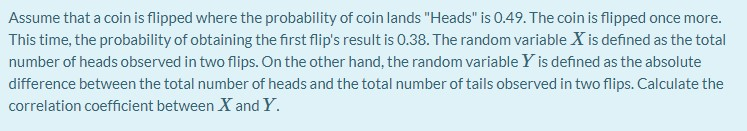Assume that a coin is flipped where the probability of coin lands "Heads" is 0.49. The coin is flipped once more. This time, the probability of obtaining the first flip's result is 0.38. The random variable X is defined as the total number of heads observed in two flips. On the other hand, the random variable Y is defined as the absolute difference between the total number of heads and the total number of tails observed in two flips. Calculate...

• ### One coin is weighted so that the probability that it lands tails up is 25%. Another...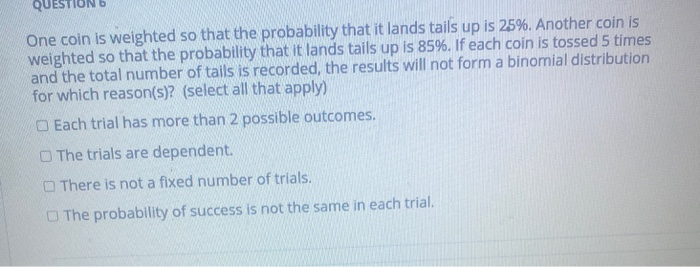One coin is weighted so that the probability that it lands tails up is 25%. Another coin is weighted so that the probability that it lands tails up is 85%. If each coin is tossed 5 times and the total number of tails is recorded, the results will not form a binomial distribution for which reason(s)? (select all that apply) Each trial has more than 2 possible outcomes. The trials are dependent There is not a fixed number of trials....

• ### Geometric Random Variables Part 1 A fair coin is flipped repeatedly until tails shows. What is...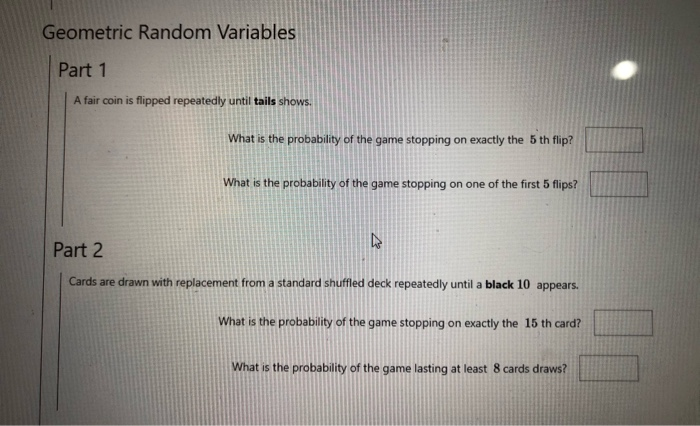Geometric Random Variables Part 1 A fair coin is flipped repeatedly until tails shows. What is the probability of the game stopping on exactly the 5 th flip? What is the probability of the game stopping on one of the first 5 flips? Part 2 Cards are drawn with replacement from a standard shuffled deck repeatedly until a black 10 appears. What is the probability of the game stopping on exactly the 15th card? What is the probability of the...

• ### Geometric Random Variables Part 1 A fair coin is flipped repeatedly until tails shows. What is...Geometric Random Variables Part 1 A fair coin is flipped repeatedly until tails shows. What is the probability of the game stopping on exactly the 5 th flip? What is the probability of the game stopping on one of the first 5 flips? Part 2 Cards are drawn with replacement from a standard shuffled deck repeatedly until a black 10 appears. What is the probability of the game stopping on exactly the 15th card? What is the probability of the...

• ### 1. A fair coin is flipped four times. Find the probability that exactly two of the...

1. A fair coin is flipped four times. Find the probability that exactly two of the flips will turn up as heads. 2. A fair coin is flipped four times. Find the probability that at least two of the flips will turn up as heads. 3. A six-sided dice is rolled twice. Find the probability that the larger of the two rolls was equal to 3. 4. A six-sided dice is rolled twice. Find the probability that the larger of...

• ### Answer part a and part b please!!! (a) What is the conditional probability that exactly four Tails appear w when a fair coin is flipped six times, given that the first flip came up Heads? (I.e. the co...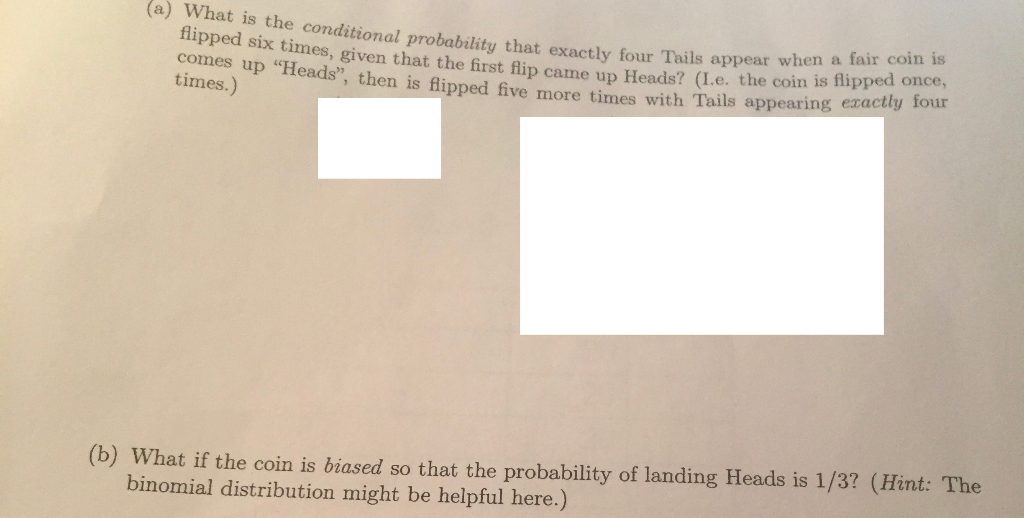Answer part a and part b please!!! (a) What is the conditional probability that exactly four Tails appear w when a fair coin is flipped six times, given that the first flip came up Heads? (I.e. the coin , then is flipped five more times with Tails appearing exactly lour times.) (b) What if the coin is biased so that the probability of landing Heads is 1/3? (Hint: The binomial distribution might be helpful here.) (a) What is the conditional...

• ### You toss a penny and observe whether it lands heads up or tails up. Suppose the...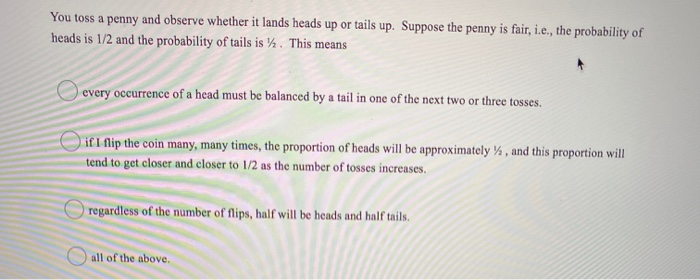You toss a penny and observe whether it lands heads up or tails up. Suppose the penny is fair, i.e., the probability of heads is 1/2 and the probability of tails is y. This means every occurrence of a head must be balanced by a tail in one of the next two or three tosses. if I flip the coin many, many times, the proportion of heads will be approximately %, and this proportion will tend to get closer and...

• ### 11.  The probability that the coin lands tails is 40%. Because the probability is not...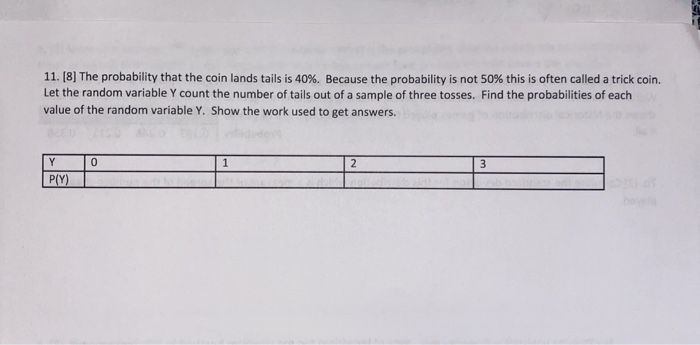11.  The probability that the coin lands tails is 40%. Because the probability is not 50% this is often called a trick coin. Let the random variable y count the number of tails out of a sample of three tosses. Find the probabilities of each value of the random variable Y. Show the work used to get answers. 0 1 2 3 Y PY)